# “Trust, but verify” SPICE model accuracy, part 3: slew rate and input clamping diodes

Previous installments of this blog post series discussed the need to verify SPICE model accuracy and how to measure common-mode rejection ratio (CMRR) and offset voltage versus common-mode voltage (VOS vs. VCM). In part 3, I’ll continue by explaining how to verify an operational amplifier (op amp) model’s slew rate, which is a large-signal output response.

Slew rate

Slew rate is defined as the maximum rate of change of an op amp’s output voltage and is typically given in volts per microsecond (V/µs). Slew rate is a type of output distortion, or nonlinearity. An amplifier in this condition is not behaving linearly where the output voltage equals the input voltage multiplied by the closed-loop gain. Instead, the op amp output voltage changes with a constant slope. This continues until the op amp corrects the difference at its input pins and the amplifier returns to a linear or small-signal operating state. For a more detailed look at slew rate, watch our TI Precision Labs – Op Amps video series on slew rate.

One of the most common ways to force an op amp into slew rate limit is to apply a large-signal input step of 100mV or greater, but slew limiting can also occur when trying to output high-amplitude signals at high frequencies. In audio applications, for example, slew limiting distorts sine waves into triangle waves, causing visible (and audible) distortion, as shown in Figure 1.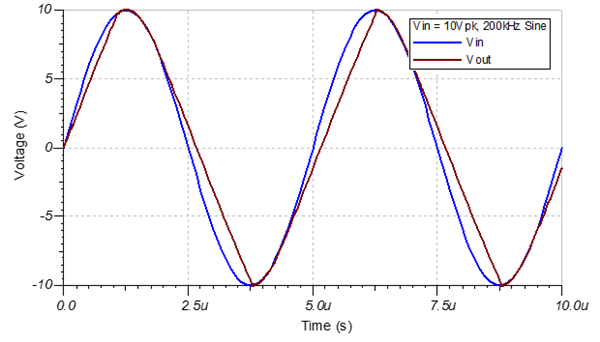Figure 1: Triangular distortion caused by slew rate limit

When using SPICE simulation for audio applications or circuits where large-signal input steps are common (such as those with multiplexers or switches), I recommend verifying the slew rate behavior of your op amp model. Figure 2 shows the recommended test circuit.

This circuit places the op amp in a unity-gain buffer configuration and applies a large-signal step to the noninverting input pin. The amplitude of the step should match the test conditions given in your specific op amp’s data sheet.

Let’s test the slew rate of the OPA196, a new e-TrimTM precision amplifier from TI, whose data sheet specifies a 10V input step test condition (Figure 3). To determine the slew rate, measure VOUT and calculate its rate of change as it transitions from 10% to 90% of the total output step.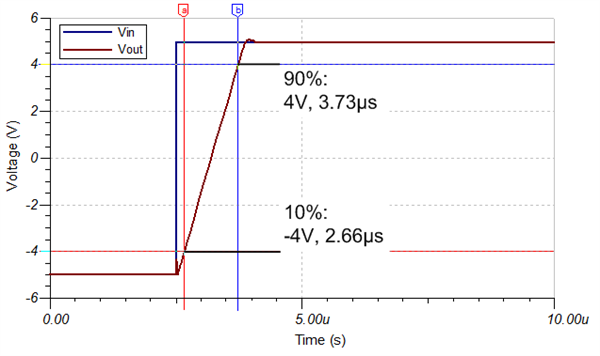Figure 3: Slew rate test results for the OPA196

Equations 1 through 3 calculate the rising slew rate for the OPA196: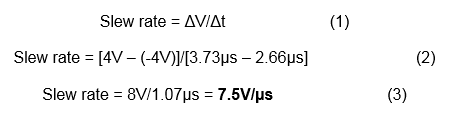where ΔV is the change in voltage and Δt is the change in time from 10% to 90% of the output transition. In this case, the simulated rising slew rate perfectly matches the data sheet spec of 7.5V/µs! You can repeat this test with a negative input step to measure the falling slew rate.

Testing amplifiers with input clamping diodes

Testing the slew rate of certain types of amplifiers requires a small tweak to the slew rate test circuit. On most bipolar, high-voltage complementary metal-oxide semiconductor (CMOS) and chopper amplifiers, clamping diodes are present across the op amp input pins. If you try to apply a large-signal step to these devices, the large differential input voltage will cause these diodes to conduct current directly from the noninverting input to the inverting input and output. The result is an incorrect slew rate measurement that’s faster than what the actual device can generate. Figure 4 shows this effect with the OPA1678, a high-voltage CMOS audio amplifier from TI.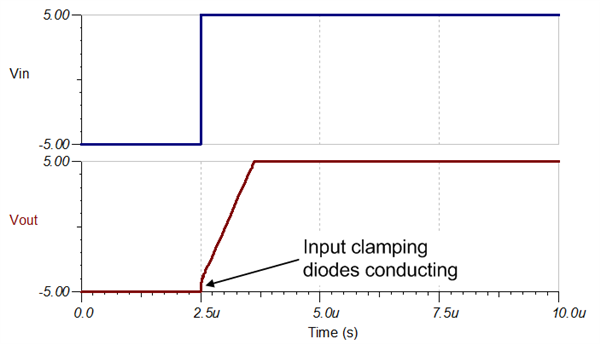Figure 4: Slew rate test results (no input current limit) for the OPA1678

This effect was not evident on the OPA196, even though it’s a high-voltage CMOS amplifier. That’s because it’s part of TI’s OPA19x family of amplifiers with multiplexer-friendly inputs. The design of the OPA19x family eliminates the need for input clamping diodes, and the amplifiers can handle large differential input voltages without them. Junction field-effect transistor (JFET)-input amplifiers such as the OPA145 also do not exhibit this issue.

To test the true slew rate of amplifiers with input clamping diodes, place a current-limiting resistor either in series with the input source or between the inverting input and the output pin. A resistance of 10kΩ does the trick for the vast majority of op amps. Figures 5 and 6 show the modified circuit and test results.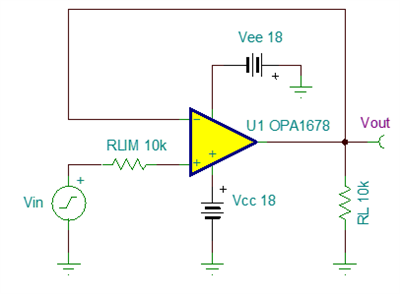Figure 5: Slew rate test circuit with input current limit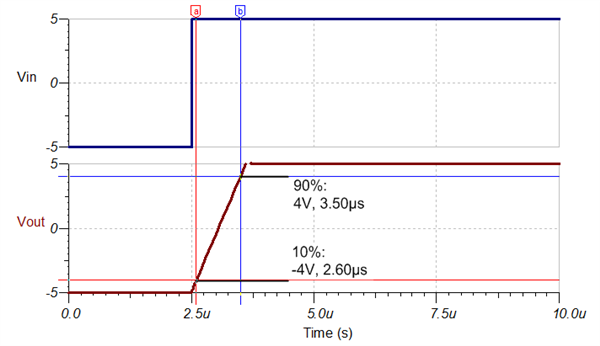Figure 6: Slew rate test results with input current limit for the OPA1678

Using Equations 1-3 once more, the rising slew rate of the OPA1678 model is 8.9V/µs – very close to the data sheet spec of 9V/µs.

As an alternative to using a large input current-limiting resistor, you can test the slew rate with the amplifier in an inverting configuration. In this case, the input and feedback resistors limit the input current through the input diodes and enable an accurate measurement.

Thanks for reading the third installment of the “Trust, but verify” blog series! In the next installment, I’ll discuss how to measure open-loop output impedance and small-signal step response to perform stability analysis. If you have any questions about simulation verification, log in and leave a comment, or visit the TI E2E™ Community Simulation Models forum.